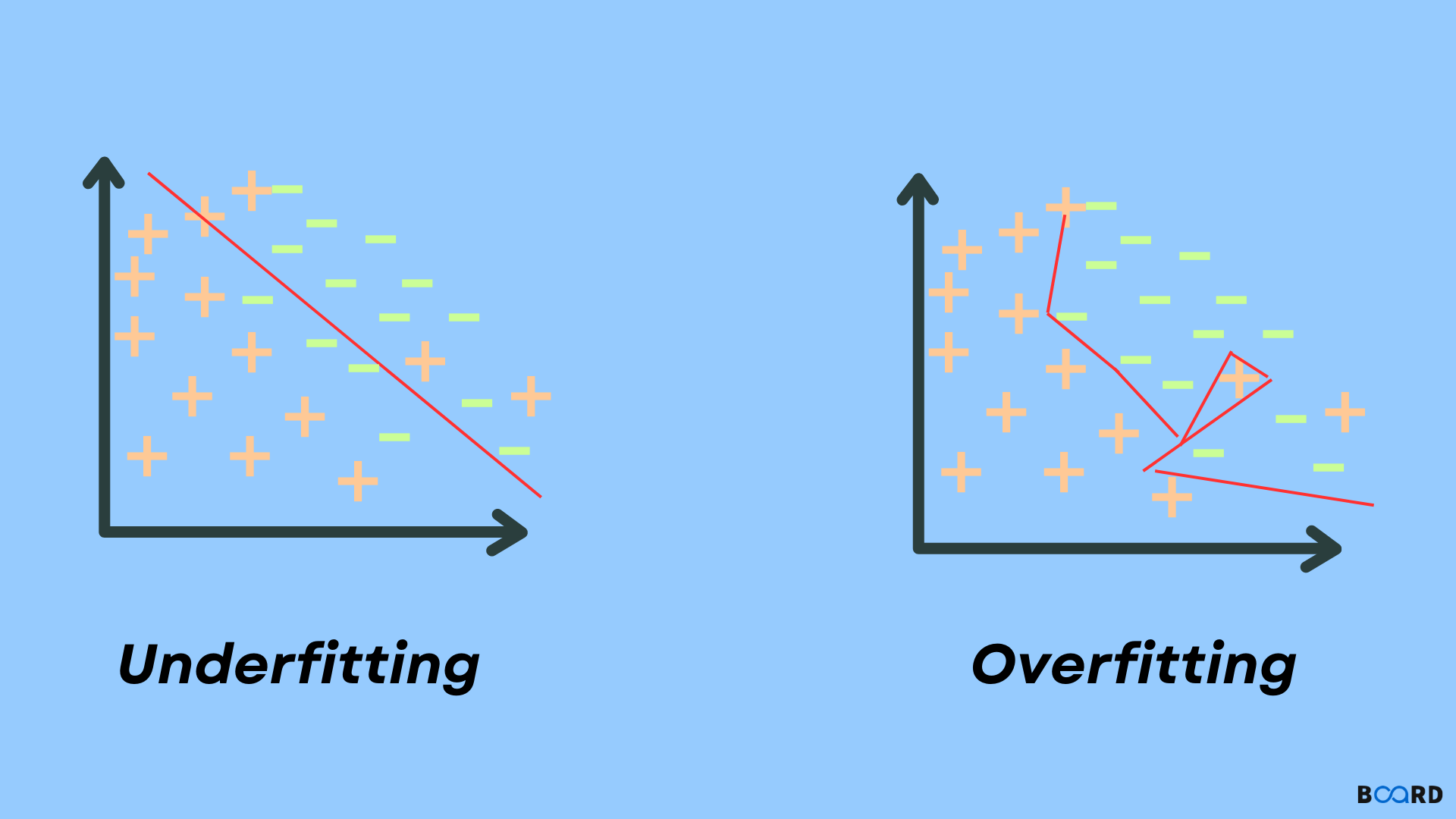# Underfitting and Overfitting in Machine Learning## Introduction

Underfitting and overfitting are 2 major issues in machine learning that stagnate the performance of a machine learning model. To aptly generalize is the primary objective of any machine learning model. Generalization means the capability of a machine learning model to adapt to the unknown set of inputs and produce a suitable output.

Thus, after being trained on a dataset, the ML model must provide precise as well as reliable results. Overfitting and underfitting hence become two important things that need to be kept an eye upon to enhance the performance of the model and to determine whether or not the model properly generalizes.

## Scope

• Basic terms and analogy.
• Overfitting in ML.
• Undefitting in ML.
• How to avoid overfitting.
• How to avoid underfitting.

• Machine learning algorithms.
• Any other unrelated ML concepts.

## Basic terms

### Signal

It is the underlying real pattern of the data that aids the ML model in learning through the data.

### Noise

It is the irrelevant non-essential data that can lower the model’s performance.

### Bias

It’s an error in prediction brought into the model due to the oversimplification of the ML algorithms.

### Variance

It is the phenomenon when the ML model performs fine with the training dataset but not with the test dataset.

## Overfitting

When an ML model attempts to capture every data point (or more than required) in a given dataset, overfitting happens. Consequently, the model begins caching the dataset’s inaccurate values and noise. Owing to these factors the accuracy, as well as the efficiency of the model, decreases. The model is then said to be overfitted and it possesses high variance and low bias.

The probability of overfitting occurring rises as the model is trained. Overfitting is a key issue in supervised learning. For a better understanding, let’s have a look at the graph of the linear regression output below:

As evident from the graph above, the model attempts to capture every data point in the scatter plot. A look at the graph may make you think that the model is efficient, but that’s not the case, because the regression model’s target is to hunt the best fit line and since there is no best fit found here, hence prediction errors are produced.

## Underfitting

When an ML model is unable to cover the underlying trend of the data, underfitting happens. The feeding of the training dataset can be halted at an early stage for avoiding overfitting in the ML model. This leads to the model not gaining sufficient learning from the training dataset. Consequently, the ML model fails in finding the best fit of the data’s dominant trend.

In underfitting, the ML model is unable to gain sufficient learning through the training dataset. Thus the accuracy of the model is decreased and unreliable predictions are produced. Note that an underfitted ML model exhibits low variance and high bias. For a better understanding, let’s have a look at the output of a linear regression model below:

## Avoiding overfitting

Following are a bunch of ways of avoiding/reducing overfitting an ML model:

• Eliminating features.
• Using more data for training.
• Stopping the training early.
• Ensembling.
• Regularization.
• Cross-validation.

## Avoiding underfitting

Following are a few ways of avoiding/reducing underfitting an ML model:

• Doing feature engineering.
• Elevating the model’s complexity.
• Incrementing the number of epochs.
• Increasing the number of features.
• Eliminating the noise from the data.

## Conclusion

• Underfitting and overfitting are 2 major issues in machine learning that stagnate the performance of a machine learning model.
• When an ML model attempts to capture every data point (or more than required) in a given dataset, overfitting happens.
• When an ML model is unable to cover the underlying trend of the data, underfitting happens